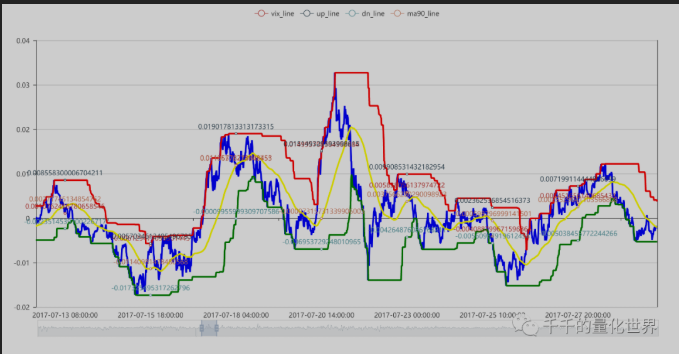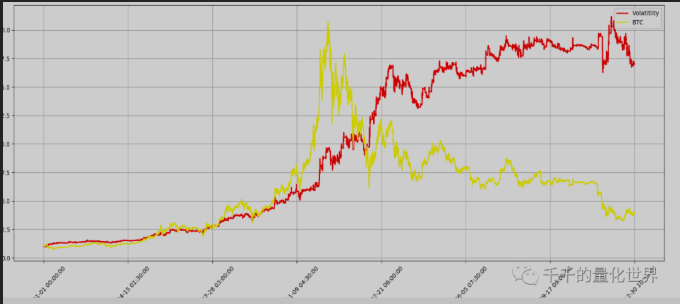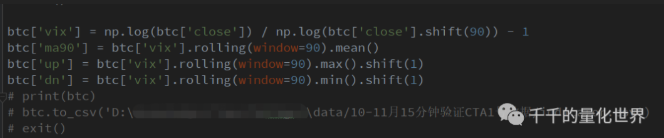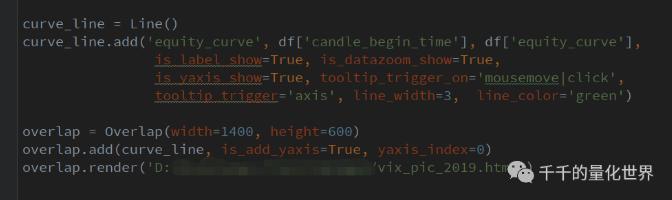# 抛硬币策略 (●'◡'●)

Author: 扁豆子, Date: 2020-04-21 17:39:52
Tags:

01

T老板说写个量化的，又没有给什么范围，着实不知道从哪里写起。那就从自己最喜欢与他人讨论的话题入手吧。量化指标与策略（即可辅助也可自动化），当然了，最后咱们还要加上一句老生常谈的话：“投资有风险，入市需谨慎”，策略只是给大家提供思路和借鉴，盈亏自负。一切使用本策略的盈亏与我本人和“千千的量化世界”公众号主体无任何关系。

0203```/*backtest
start: 2020-01-20 00:00:00
end: 2021-01-19 23:59:00
period: 15m
basePeriod: 5m
exchanges: [{"eid":"Futures_BitMEX","currency":"XBT_USD","fee":[0.008,0.1]}]
args: [["st",0.1]]
*/

// 初始化
exchange.SetContractType('XBTUSD')
_CDelay(100)
// 止盈止损
var TP_status = false // 是否触发追踪止盈
var TP_HH = 0
var TP_LL = 0
var B = 1

// 获取交易所信息
function UpdateInfo() {
account = exchange.GetAccount()
pos = exchange.GetPosition()
records = exchange.GetRecords()
ticker = exchange.GetTicker()
}

// 定制本次盈亏
function Onept() {
// 更新用户信息
UpdateInfo()
// 如果现在余额 大于 之前的余额, 那么 盈利次数+1, 且pt_1设为现在余额
if (account.Stocks - pt_1 > 0) {
pt_times = pt_times + 1
Log('这回赚钱啦~~~~ (＾Ｕ＾)ノ~ＹＯ', account.Stocks - pt_1)
B = 1
pt_1 = account.Stocks
}
// 如果现在余额 小于 之前的余额, 那么 亏损次数+1, 且pt_1设为现在余额
if (account.Stocks - pt_1 < 0) {
st_times = st_times + 1
Log('这回亏掉了.... /(ㄒoㄒ)/~~', account.Stocks - pt_1)
B = B * 1.618
pt_1 = account.Stocks
}
}

// 画线
function PlotMA_Kline(records) {
\$.PlotRecords(records, "K")
}

// 追踪止盈 初始%, 追踪U
function TP() {
var TP_first_long = pos.Price + tp_first * ticker.Last
var TP_trailing_long = TP_HH - trailing_tp * ticker.Last
var TP_first_short = pos.Price - tp_first * ticker.Last
var TP_trailing_short = TP_LL + trailing_tp * ticker.Last
// 当多仓时, 现价大于开仓+初始止赢价 -> 触发追踪止盈
if ((pos.Type == 0) && (ticker.Last > TP_first_long)) {
// Log('当多仓时, 现价大于开仓+初始止赢价 -> 触发追踪止盈', TP_HH)
TP_status = true
// 触发追踪止盈, 未初始化开仓最大价格 -> 开仓后最大价格更新为现价
if (TP_status === true && TP_HH == 0) {
Log('触发追踪止盈, 未初始化开仓最大价格 -> 开仓后最大价格更新为现价', TP_HH)
TP_HH = ticker.Last
}
// 触发追踪止盈, 已有开仓后最大价格, 现价大于开仓后最大价格 -> 开仓后最大价格更新为现价
else if (TP_status === true && TP_HH != 0 && ticker.Last > TP_HH) {
Log('触发追踪止盈, 已有开仓后最大价格, 现价大于开仓后最大价格 -> 开仓后最大价格更新为现价', TP_HH)
TP_HH = ticker.Last
}
// 触发追踪止盈, 已有开仓后最大价格, 现价小于 (开仓后最大价格减 - 回撤USD) -> 开空平仓止盈
else if (TP_status === true && TP_HH != 0 && ticker.Last < TP_trailing_long) {
Log('触发追踪止盈, 已有开仓后最大价格, 现价小于 (开仓后最大价格减 - 回撤USD) -> 开空平仓止盈', TP_HH)
exchange.Sell(ticker.Buy, pos.Amount, "在" + ticker.Last + "止赢平多仓!! 开仓价格: " + pos.Price + "数量: " + pos.Amount)
\$.PlotFlag(new Date().getTime(), 'Sell', 'PT_BK' + ticker.Sell)
Onept()
TP_status = false
TP_HH = 0
}
}
// 当空仓时, 现价小于开仓-初始止赢价 -> 触发追踪止盈
else if ((pos.Type == 1) && (ticker.Last < TP_first_short)) {
// Log('当空仓时, 现价小于开仓-初始止赢价 -> 触发追踪止盈', TP_LL)
TP_status = true
// 触发追踪止盈, 未初始化开仓最大价格 -> 开仓后最小价格更新为现价
if (TP_status === true && TP_LL == 0) {
Log('触发追踪止盈, 未初始化开仓最大价格 -> 开仓后最小价格更新为现价', TP_LL)
TP_LL = ticker.Last
}
// 触发追踪止盈, 已有开仓后最小价格, 现价小于开仓后最小价格 -> 开仓后最小价格更新为现价
else if (TP_status === true && TP_LL != 0 && ticker.Last < TP_LL) {
Log('触发追踪止盈, 已有开仓后最小价格, 现价小于开仓后最小价格 -> 开仓后最小价格更新为现价', TP_LL)
TP_LL = ticker.Last
}
// 触发追踪止盈, 已有开仓后最小价格, 现价大于 (开仓后最小价格减 + 回撤USD) -> 开多平仓止盈
else if (TP_status === true && TP_LL != 0 && ticker.Last > TP_trailing_short) {
Log('触发追踪止盈, 已有开仓后最小价格, 现价大于 (开仓后最小价格减 + 回撤USD) -> 开多平仓止盈', TP_LL)
exchange.SetDirection("closesell")
exchange.Buy(ticker.Sell, pos.Amount, "在" + ticker.Last + "止赢平空仓!! 开仓价格: " + pos.Price + "数量: " + pos.Amount)
\$.PlotFlag(new Date().getTime(), 'Buy', 'PT_SK' + ticker.Sell)
Onept()
TP_status = false
TP_LL = 0
}
}
}

// 止损 %
function Stoploss() {
// 当多仓时, 现价小于开仓-止损价, 做空平多
if ((pos.Type == 0) && (ticker.Last < pos.Price - st * ticker.Last)) {
Log('当多仓时, 现价小于开仓-止损价, 做空平多')
exchange.Sell(ticker.Buy, pos.Amount, "在" + ticker.Last + "止损平多仓!! 开仓价格: " + pos.Price + "数量: " + pos.Amount)
\$.PlotFlag(new Date().getTime(), 'Sell', 'ST_BK' + ticker.Buy)
Onept()
}
// 当空仓时, 现价大于开仓+止损价, 做多平空
else if ((pos.Type == 1) && (ticker.Last > pos.Price + st * ticker.Last)) {
Log('当空仓时, 现价大于开仓+止损价, 做多平空')
exchange.SetDirection("closesell")
exchange.Buy(ticker.Sell, pos.Amount, "在" + ticker.Last + "止损平空仓!! 开仓价格: " + pos.Price + "数量: " + pos.Amount)
\$.PlotFlag(new Date().getTime(), 'Buy', 'ST_SK' + ticker.Sell)
Onept()
}
}

// 计算凯利公式 仓位
function PriceAmount() {
// 赢可以赢多少
y = tp_first
// 输会输多少
s = st
//赔率
b = y / s
// 赢的概率
if (total_times < 10) {
p = 0.382
} else {
p = pt_times / total_times
}
// 输的概率
q = 1 - p
// 凯莉公式
f = (b * p - q) / b
// 限制B最大值
if (B > 16.18) {
B = 16.18
}
//Amount = _N(Math.abs(f) * account.Stocks * ticker.Last * B, 0)
Amount = _N(0.618 * account.Stocks * ticker.Last, 0)
//Log(Amount)
}

// 交易逻辑
function onTick() {
// 获取均匀分布 0-9 随机数
ToTheMoon = Math.floor(Math.random() * 10)
// 无仓位时
if (pos.length == 0) {
// Long
if (ToTheMoon > 5) {
\$.PlotFlag(new Date().getTime(), 'Buy', 'BK' + ticker.Sell)
total_times = total_times + 1
}
// Short
if (ToTheMoon < 4) {
exchange.SetDirection("sell")
\$.PlotFlag(new Date().getTime(), 'Sell', 'SK' + ticker.Buy)
total_times = total_times + 1
}
}
// 多仓时
if (pos.length > 0 && pos.Type == 0) {
// 平多
if (ToTheMoon < 1) {
\$.PlotFlag(new Date().getTime(), 'Sell', 'PBK')
Onept()
}
}
// 空仓时
if (pos.length > 0 && pos.Type == 1) {
// 平空
if (ToTheMoon > 8) {
exchange.SetDirection("closesell")
Onept()
}
}
}

function main() {
UpdateInfo()
// 统计
pt_1 = account.Stocks
total_times = 0
pt_times = 0
st_times = 0
while (1) {
UpdateInfo()
PriceAmount()
onTick()
PlotMA_Kline(records)
if (pos.length > 0) {
TP()
}
if (pos.length > 0) {
Stoploss()
}
LogStatus("总余额: " + _N(ticker.Last * account.Stocks, 2), " 下单量: " + Amount, " 下单倍数: " + B, " ToTheMoon: " + ToTheMoon, " 下单量比: " + _N(Amount * 100 / _N(ticker.Last * account.Stocks, 2), 2), "% 胜率: " + _N(p * 100, 2), "%", total_times, pos)
}
}
```

More